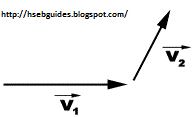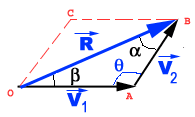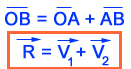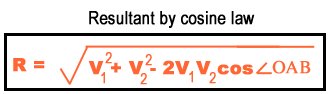## TyroCity

Physics XI Notes for Physics Notes

Posted on • Updated on

# Parallelogram Law Of Vector Addition

According to the parallelogram law of vector addition:
“If two vector quantities are represented by two adjacent sides or a parallelogram then the diagonal of parallelogram will be equal to the resultant of these two vectors.”

Explanationparallelogram of these vectors is :According to parallelogram law:Magnitude Of resultant Vector
Magnitude of resultant vector can be determined by using either sine law or cosine law.